# PSEB 11th Class Maths Solutions Chapter 9 Sequences and Series Ex 9.2

Punjab State Board PSEB 11th Class Maths Book Solutions Chapter 9 Sequences and Series Ex 9.2 Textbook Exercise Questions and Answers.

## PSEB Solutions for Class 11 Maths Chapter 9 Sequences and Series Ex 9.2

Question 1.
Find the sum of odd integers from 1 to 2001.
The odd integers from 1 to 2001 are 1, 3, 5, … 1999, 2001.
This sequence forms an A.P.
Here, first term, a = 1
Common difference, d = 2
Here, a + (n – 1) d = 2001
⇒ 1 + (n -1) (2) = 2001
⇒ 2n – 2 = 2000
⇒ n = 1001
Sn = $$\frac{n}{2}$$ [2a + (n – 1)d]
= $$\frac{1001}{2}$$ [2 × 1 + (1001 – 1) × 2]
= $$\frac{1001}{2}$$ [2 + 1000 x 2]
= $$\frac{1001}{2}$$ × 2002
= 1001 × 1001
= 1002001
Thus, the sum of odd numbers from 1 to 2001 is 1002001.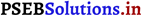Question 2.
Find the sum of all natural numbers lying between 100 and 1000, which are multiples of 5.
The natural numbers lying between 100 and 1000, which are multiples of 5 are 105, 110, …, 995.
Here, a = 105 and d = 5
a + (n – 1) d = 995
⇒ 105 + (n -1)5 = 995
⇒ (n – 1)5 = 995 – 105 = 890
⇒ n – 1 = 178
⇒ n = 179
Sn = $$\frac{179}{2}$$ [2 (105) + (179 – 1) (5)]
= $$\frac{179}{2}$$ [2 (105) + (178) (5)]
= 179 [105 + (89)5]
= (179) (105 + 445)
= (179) (550) = 98450
Thus, the sum of all natural numbers lying between 100 and 1000, which are multiples of 5 is 98450.

Question 3.
In an A.P. the first term is 2 and the sum of the first five terms is one-fourth of the next five terms. Show that 20th term is – 112.
Let the first term of given AP be a and common difference be d.
We have, T1 = a = 2
T1 + T2 + T3 + T4 + T5 = [T6 + T7 + T8 + T9 + T10]
Sum of 5 terms, where first term is a = $$\frac{1}{4}$$ × sum of 5 terms, where first term is (a + 5d)
⇒ $$\frac{5}{2}$$ [2a + (5 – 1) d] = $$\frac{1}{4}$$ × $$\frac{5}{2}$$ [2(a + 5d) + (5 – 1)d]
[∵ Sn = $$\frac{n}{2}$$ [2a + (n – 1)d]]
$$\frac{5}{2}$$ [2a + 4d] = $$\frac{1}{4}$$ × $$\frac{5}{2}$$ [2a + 10d + 4d]
$$\frac{5}{2}$$ [2a + 4d] = $$\frac{1}{4}$$ × $$\frac{5}{2}$$ [2a + 14d]
⇒ 2a + 4d = $$\frac{1}{4}$$ [2a + 14d]
2(2) + 4d = $$\frac{1}{4}$$ [2 . (2) + 14d] [put a = 2]
4 + 4d = $$\frac{1}{4}$$ [4 + 14d]
16 + 16d = 4 + 14d
16d – 14d = 4 – 16
2d = – 12
d = – 6
T20 = a + (20 – 1) d
= 2 + 19 × (- 6)
= 2 – 114 = – 112
Hence proved.Question 4.
How many terms of the A.P. – 6, – $$\frac{11}{2}$$, – 5, … are needed to give the sum – 25?
Let the sum of a terms of the given A.P. be -25.
It is known that, Sn = $$\frac{n}{2}$$ [2a + (n – 1) d],
where n = number of terms, a = first term, and d common difference
Here, a = – 6
d = $$-\frac{11}{2}+6=\frac{-11+12}{2}=\frac{1}{2}$$
Therefore, we obtain
– 25 = $$\frac{n}{2}\left[2 \times(-6)+(n-1)\left(\frac{1}{2}\right)\right]$$
– 50 = n $$\left[-\frac{25}{2}+\frac{n}{2}\right]$$
⇒ – 100 = n (- 25 + n)
⇒ n2 – 25n + 100 = 0
⇒ n2 – 5n – 20n + 100 = 0
⇒ n (n – 5) – 20 (n – 5) = 0
⇒ n = 20 or 5.

Question 5.
In an A.P., if pth term is $$\frac{1}{q}$$ and qth term is $$\frac{1}{p}$$, prove that the sum of first pq terms is $$\frac{1}{2}$$ (pq + 1), where pq ≠ q.
It is known that the general term of an A.P. is
an = a + (n – 1) d
According to the given information,
pth term = ap
= a + (p – 1)d = $$\frac{1}{q}$$ …………..(i)
qth term = aq
= a + (q – 1)d = $$\frac{1}{p}$$ …………….(ii)
Subtracting eq. (ii) from eq. (i), we obtain
(p – 1) d – (q – 1) d = $$\frac{1}{q}-\frac{1}{p}$$
(p – 1 – q + 1) d = $$\frac{p-q}{p q}$$
⇒ (p – q) d = $$\frac{p-q}{p q}$$
d = $$\frac{1}{p q}$$
Putting the value of d in eq. (i), we obtain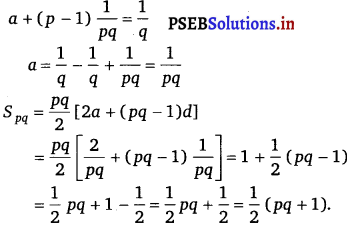Thus, the sum of first pq terms of the A.P. is $$\frac{1}{2}$$ (pq + 1).Question 6.
If the sum of a certain number, of terms of the A.P. 25, 22, 19, …is 116. Find the last term.
Let the sum of n terms of the given A.P. be 116.
Sn = $$\frac{n}{2}$$ [2a + (n – 1)d]
Here, a = 25 and d = 22 – 25 = – 3
Sn = $$\frac{n}{2}$$ [2 × 25 + (n – 1) (- 3)]
⇒ 116 = $$\frac{n}{2}$$ [50 – 3n + 3]
⇒ 232 = n(53 – 3n) = 53n – 3n2
3n2 – 24n – 29n + 232 = 0
3n (n – 8) – 29 (n – 8) = 0
(n – 8) (3n – 29) = 0
n = 8 or n = $$\frac{29}{3}$$
However, n cannot be equal to $$\frac{29}{3}$$.
Therefore, n = 8
∴ a8 = Last term = a + (n -1) d = 25 + (8 – 1) (- 3)
= 25 + (7) (- 3) = 25 – 21 = 4
Thus, the last term of the A.P. is 4.

Question 7.
Find the sum of n terms of the A.P., whose kth term is 5k + 1.
It is given that the kth term of the A.P. is 5k + 1
kth term = ak = a + (k – 1) d
a + (k – 1) d = 5k +1
a + kd – d = 5k + 1
Comparing the coefficient of k, we obtain d = 5
a – d = 1
⇒ a – 5 = 1
⇒ a = 6
Sn = $$\frac{n}{2}$$ [2a + (n – 1) d]
= $$\frac{n}{2}$$ [2 (6) + (n – 1) (5)]
= $$\frac{n}{2}$$ [12n + 5n – 5]
= $$\frac{n}{2}$$ (5n + 7).Question 8.
If the sum of n terms of an A.P. is (pn + qn 2), where p and q are constants, find the common difference.
It is known that, Sn = $$\frac{n}{2}$$ [2a + (n – 1)d]
According to the given condition,
$$\frac{n}{2}$$ [2a + (n – 1 )d] = pn + qn2
$$\frac{n}{2}$$ [2a + nd – d] = pn + qn2

na + n2 $$\frac{d}{2}$$ – n . $$\frac{d}{2}$$ = pn + qn2
Comparing the coefficient of n2 on both sides, we obtain
$$\frac{d}{2}$$ = q
d = 2q
Thus, the common difference of the A.P. is 2q.

Question 9.
The sums of n terms of two arithmetic progressions are in the ratio 5n + 4 : 9n + 6. Find the ratio of their 18th terms.
Let a1, a2 and d1, d2 be the first terms and the common difference of the first and second arithmetic progression respectively.
According to the given condition,
Sum of n terms of first A.P. 5n + 4
Sum of n terms of second A.P. 9n + 6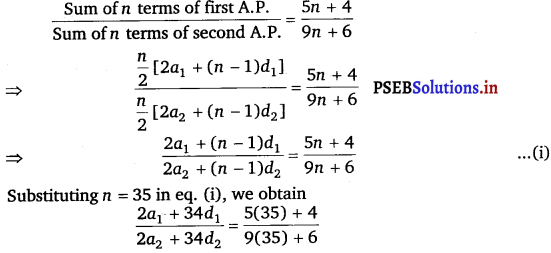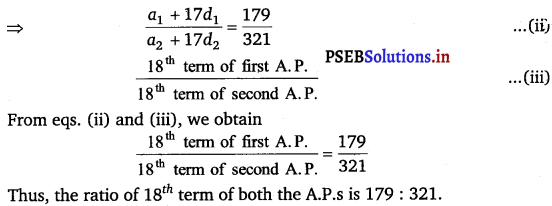Question 10.
If the sum of first p terms of an A.P. is equal to the sum of the first q terms, then find sum of the first (p + q) terms.
Let a and b be the first term and the common difference of the A.P. respectively.
Here, Sp = $$\frac{p}{2}$$ [2a + (p – 1)d]
Sq = $$\frac{q}{2}$$ [2a + (q – 1 )d]
According to the given condition,
$$\frac{p}{2}$$ [2a + (p – 1) d] = $$\frac{q}{2}$$ [2a + (q – 1) d]
p [2a + (p – 1) d] = q [2a + (n – 1) d]
2ap + pd (p – 1) d = 2aq + qd (q – 1) d
2a (p – q) + d[p(p – 1) – q (q – 1)] = 0
2a (p – q) + d[p2 – p – q2 + q] = 0
2a (p – q) + d [(p – q) (p + q) – (p – q)] = 0
2a (p – q) + d [(p – q) (p + q – 1)] = 0
2a + d (p + q – 1) = 0
d = $$\frac{-2 a}{p+q-1}$$ ……………..(i)

∴ Sp + q = $$\frac{p+q}{2}$$ [2a + (p + q – 1) d]
Sp + q = $$\frac{p+q}{2}\left[2 a+(p+q-1)\left(\frac{-2 a}{p+q-1}\right)\right]$$ [from eq. (i)]
= $$\frac{p+q}{2}$$ [2a – 2a] = 0
Thus, the sum of the first (p + q) terms of the A.P. is 0.

Question 11.
Sum of the first p, q and r terms of an A.P. are a, b and c, respectively.
Prove that $$\frac{a}{p}$$ (q – r) + $$\frac{b}{q}$$ (r – p) + $$\frac{c}{r}$$ (p – q) = 0
Given that, Sp = a, Sq = b, Sc = r
Let A be the first term and d be the common difference. Then,
Sp = $$\frac{p}{2}$$ [2A + (p – 1) d] = a
2A + (p – 1) d = $$\frac{2 a}{p}$$ ……………(i)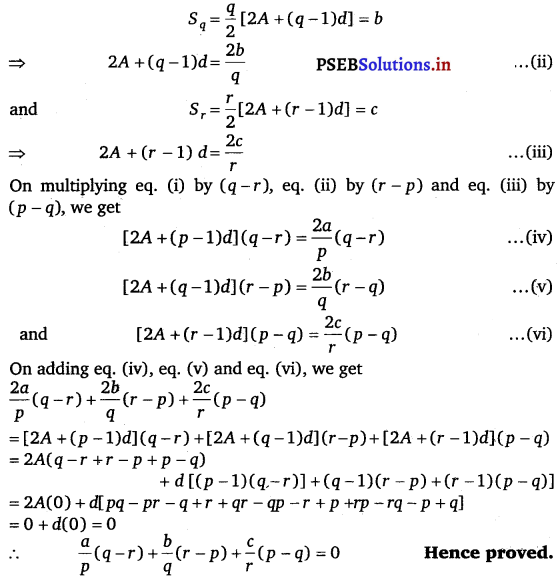Question 12.
The ratio of the sums of m and n terms of an A.P. is m2 : n2. Show that the ratio of mth and nth term is (2m – 1): (2n – 1).
Let the first term “be a and common difference be d.
Thus, Sm = $$\frac{m}{2}$$ [2a + (m – 1)d] and Sn = $$\frac{n}{2}$$ [2a + (n – 1)d]
According to the given condition.
$$\frac{S_{m}}{S_{n}}=\frac{m^{2}}{n^{2}}$$

$$\frac{\frac{m}{2}[2 a+(m-1) d]}{\frac{n}{2}[2 a+(n-1) d]}=\frac{m^{2}}{n^{2}} \Rightarrow \frac{2 a+(m-1) d}{2 a+(n-1) d}=\frac{m}{n}$$
2an + (mn – n) d = 2am + (mn – m)d
2an – 2am = (mn – m – mn + n)d
2a (n – m) = d (n – m)
⇒ d = 2a .
Tm = a + (m – 1) d = a + (m – 1) 2a
Tm = a + 2am – 2a
Tm = 2am – a
⇒ Tm = a (2m – 1) ……………..(i)
Also, Tn = a (2n – 1) ………………(ii)
On dividing eQuestion (i) by eQuestion (ii), we get
$$\frac{T_{m}}{T_{n}}=\frac{a(2 m-1)}{a(2 n-1)}=\frac{2 m-1}{2 n-1}$$
Hence proved.

Question 13.
If the sum of n terms of A.P. is 3n2 + 5 n and its mth term is 164, find the value of m.
Let a and b be the first term and the common difference of the A.P., respectively
am = a + (m – 1) d = 164 ……………(i)
Sum of n terms
Here, $$\frac{n}{2}$$ [2a + nd – d] = 3n2 + 5n
$$n a+\frac{n^{2} d}{2}-\frac{n d}{2}=3 n^{2}+5 n$$

$$\frac{n^{2} d}{2}+n\left(a-\frac{d}{2}\right)$$ = 3n2 + 5n

Comparing the coefficient of n2 on both sides, we obtain
$$\frac{d}{2}$$ = 3
⇒ d2 = 2 × 3 = 6
Comparing the coefficient of n on both sides, we obtain
a – $$\frac{d}{2}$$ = 5
a – $$\frac{6}{2}$$ = 5
a = 5 + 3 = 8
Therefore, from eq. (i), we obtain
8 + (m – 1) 6 = 164
⇒ (m – 1) 6 = 164 – 8 = 156
⇒ m – 1 = 26
⇒ m = 27
Thus the value of m is 27.Question 14.
Insert five numbers between 8 and 26 such that the resulting sequence is an A.P.
Let A1, A2, A3, A4 and A5 be five numbers between 8 and 26 such that 8, A1, A2, A3, A4, A5, 26 is an A.P.
Here, a = 8, b = 26, n = 7
Therefore, 26 = 8 + (7 – 1)d
6d = 26 – 8 = 18
d = 3
A1 = a + d = 8 + 3 = 11
A2 = a + 2d = 8 + 2 × 3 = 8 + 6 = 14
A3 = a + 3d = 8 + 3 × 3 = 8 + 9 = 17
A4 = a + 4d = 8 + 4 × 3 = 8 + 12 = 20
A5 = a + 5d = 8 + 5 × 3 = 8 + 15 = 23.
Thus, the required five numbers between 8 and 26 are 11, 14, 17, 20 and 23.

Question 15.
If $$\frac{a^{n}+b^{n}}{a^{n-1}+b^{n-1}}$$ is the A.M. etween a and b, then find the value of n.
We know that, AM between a and b is $$\frac{a+b}{2}$$.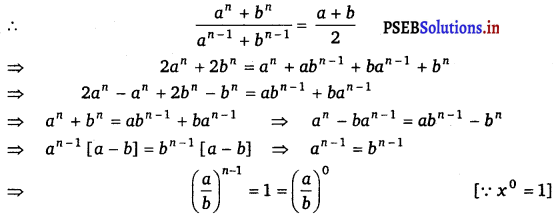On comparing the exponential powers, we get
n – 1 = 0
⇒ n = 1.Question 16.
Between 1 and 31, m numbers have been inserted in such a way that the resulting sequence is an A.P. and the ratio of 7th and (m – 1)th numbers is 5 : 9. Find the value of m.
Let A1, A2, A3, A4, … Am be m A.Ms.between 1 and 31.
Therefore, 1, A1, A2, A3, ……… Am, 31 are in A.P.
Let d be the common difference of AP.
Here, the total number of terms is m + 2 and Tm + 2 = 31
⇒ 1 + (m + 2 – 1) d = 31
⇒ (m + 1) d = 30
⇒ d = $$\frac{30}{m + 1}$$ ……………….(i)
A7 = T8 = a + 7d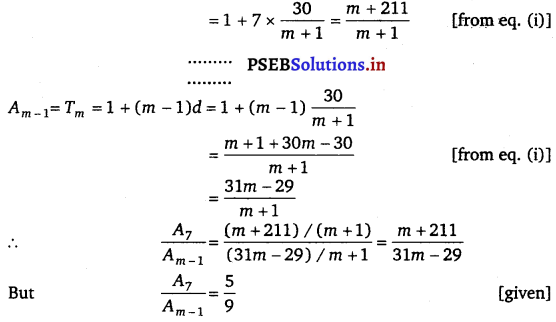$$\frac{m+211}{31 m-29}=\frac{5}{9}$$
⇒ 9m + 1899 = 155m – 145
⇒ 146m = 2044
⇒ m = $$\frac{2044}{146}$$ = 14
∴ m = 14Question 17.
A man starts repaying a loan as first installment of Rs. 100. If he increases the installment by Rs. 5 every month, what amount he will pay in the 30th installment?
The first installment of the loan is Rs. 100.
The second installment of the loan is Rs. 105 and so on.
The amount that the man repays every month forms an A.P.
The A.P. is 100, 105, 110, ………..
First term, a = 100 Common difference, d = 5
A30 = a +(30 – 1)d
= 100 + (29) (5) = 100 + 145 = 245.
Thus, the amount to be paid in the 30th installment is Rs. 245.Question 18.
The difference between any two consecutive interior angles of a polygon is 5°. If the smallest angle is 120°, find the number of the sides of ihe polygon.
The angles of the polygon will form an A.P. with common difference d as 5° and first term a as 120°.
It is known that the sum of all angles of a polygon with n sides is 180° (n – 2).
∵ Sn = 180° (n – 2)
$$\frac{n}{2}$$ [2a + (n -1) d] = 180° (n – 2)
$$\frac{n}{2}$$ [240° + (n – 1) 5°] = 180 (n – 2)
n [240 + (n – 1) 5] = 360 (n – 2)
240n + 5n2 – 5n = 360n – 720
⇒ 5n2 + 235n – 360n + 720 = 0
⇒ 5n2 – 125n + 720 = 0
⇒ n2 – 25n + 144 = 0
⇒ n2 – 16n – 9n + 144 = 0
⇒ n (n – 16) – 9 (n – 16) = 0
⇒ (n – 9) (n – 16) = 0
⇒ n = 9 or 16.